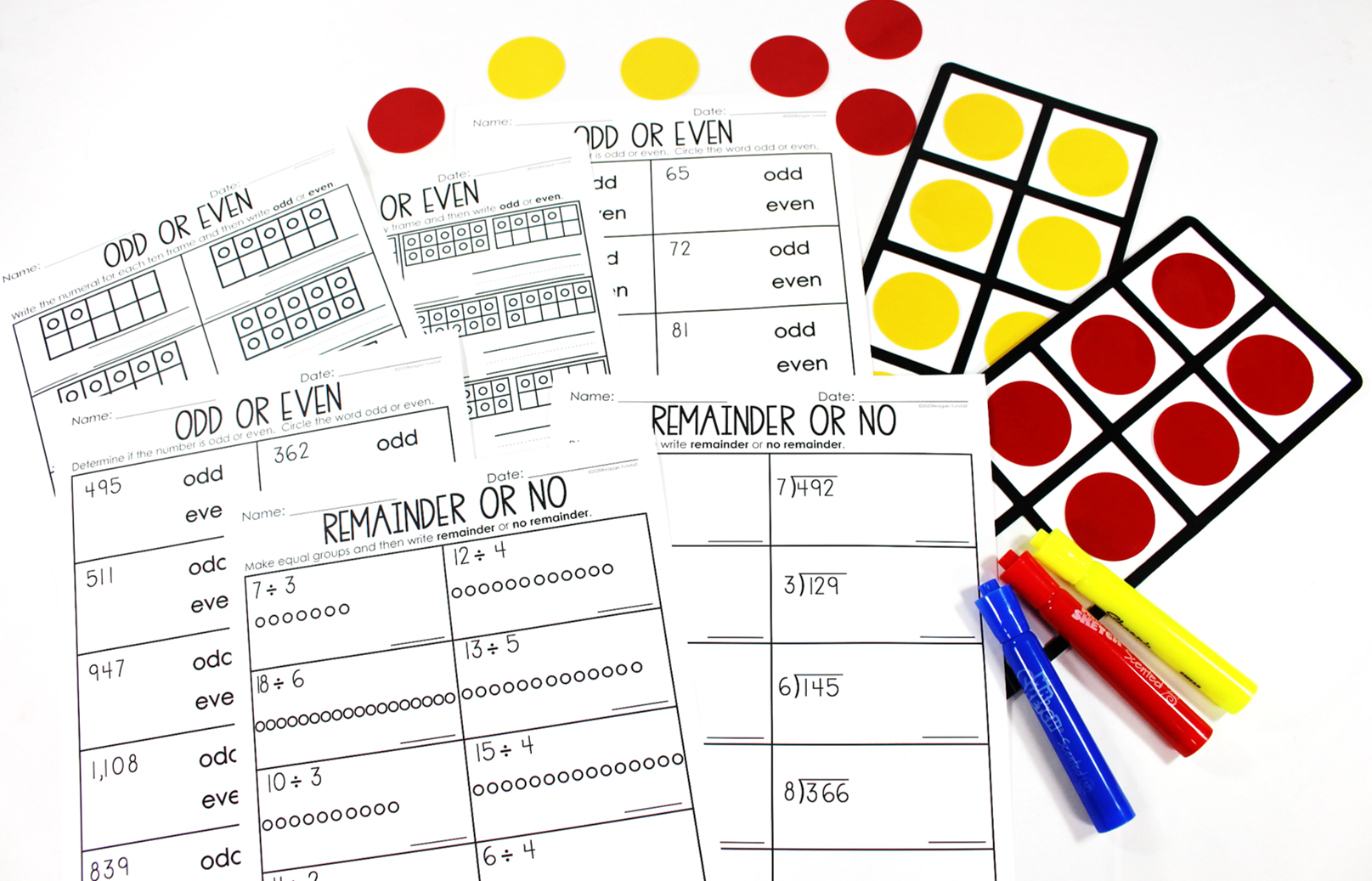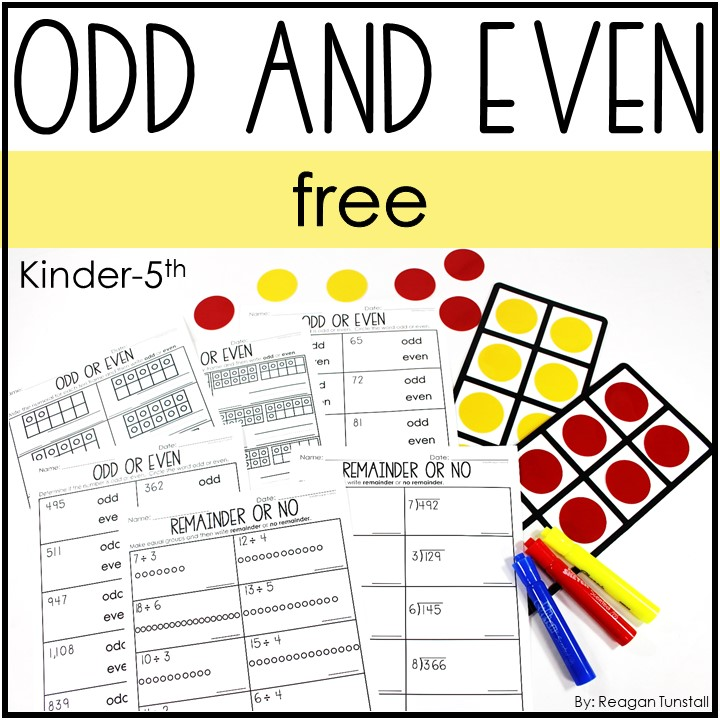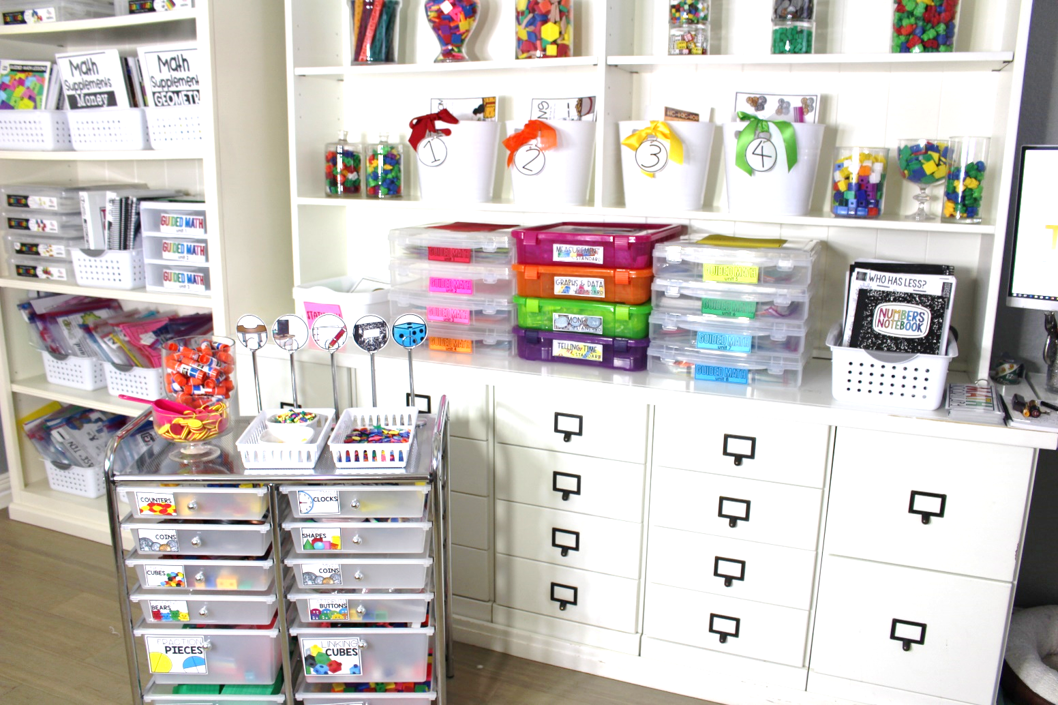# The Importance of Understanding Odd and Even in K-5## It all started with a comment

Recently we received a comment about how odd and even was taught in our digital guided math resource series.  In this comment, the happy teacher explained how much her students understood the concept of odd and even because of the use of ten frames. Using a ten frame allows students to take a given number and manipulate the dots representing that number.  When students shift and move dots representing our number value into pairs they can see if there are just pairs within the number (divisible by 2) or whether there is an oddball (not divisible by 2).

### Odd and Even in K-5

Like many of us do, we use skip counting and number lines to introduce and teach odd and even.  Our lessons go as far as making cute characters from books and doing sorts of numbers.  We have students make a quick sketch or use manipulatives to pair up partners and oddballs.  These are valuable lessons.  They help introduce and allow students to practice the concept.

With the goal being for students to be able to mentally organize information on whether that number is divisible by two or whether there would be a remainder, inserting a ten frame into the mix adds a layer of organization that the brain can connect to.  This organization of the number ten and the combinations within it is the key factor in why this is such a successful method for teaching odd and even.Inherently students see numbers within ten and can take a number such as 5 and manipulate it into 2 sets of 2 with a remainder of 1.  This is the building block of division.  Through the use of this model, students can more easily reference and understand divisibility. The more time spent in the concrete, manipulating numbers, the more fluid the mathematician will be flexibly working with numbers.  For example, students given 15÷3 can draw on their experiences with a twenty frame and visually see 3 sets of 5 within that framework.

Here’s an odd and even freebie to add to your mix of teaching!

This free resource includes:

• Odd and Even to 10
• Odd and Even to 20
• Odd and Even to 120
• Odd and Even to 1,200
• Remainder or No Simple Division
• Remainder or No DivisionCome read more about how I run math in my classroom!## One Comment

1.Ramya Ravindra Barithaya says:

Love the article